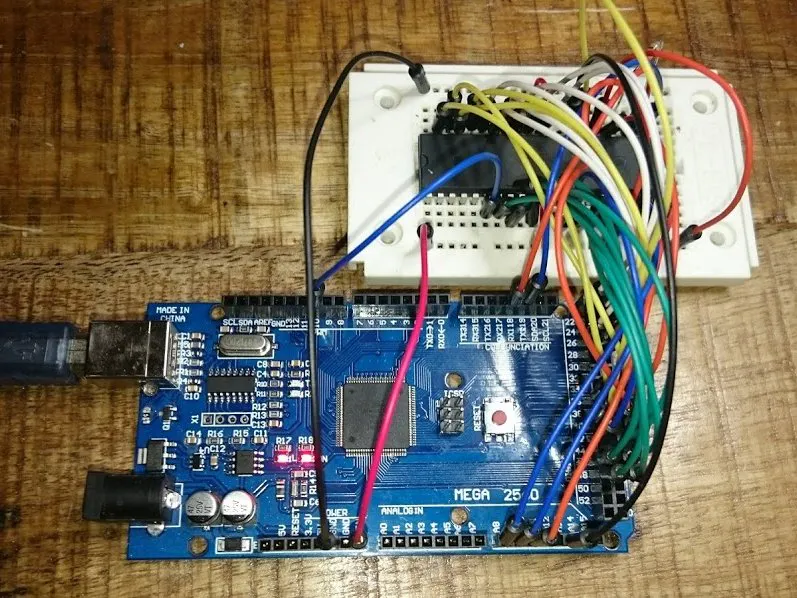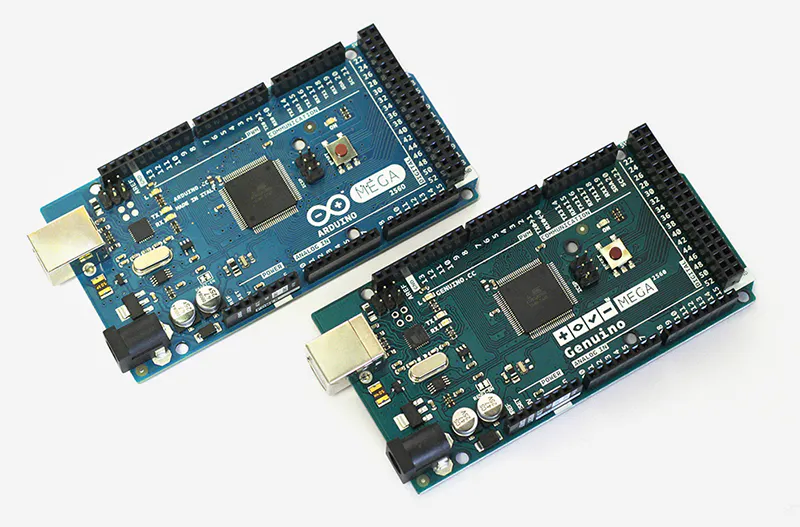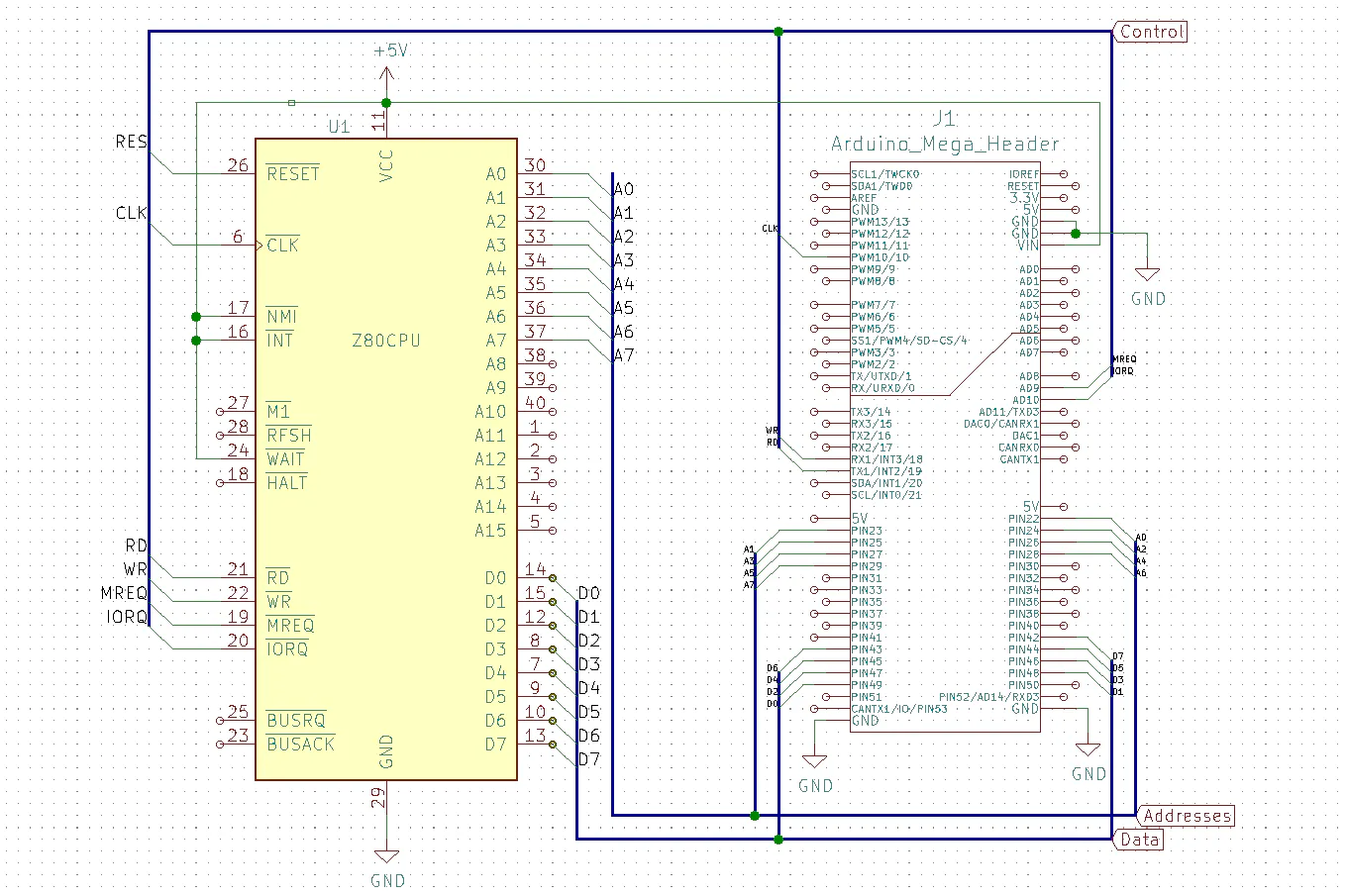# Retro Computing with Arduino Mega and a Z80 processor

Brought to the marked in 1976, Zilog's Z80 was the most widespread processor in the '80s until the 2000s. Let's bring it to life again!## Things used in this project

### Hardware componentsArduino Mega 2560 & Genuino Mega 2560
×1
 Z80 Processor
×1×1Jumper wires (generic)
×1

### Software apps and online servicesArduino IDE

## Schematics

### Schematic## Code

### z80-test.ino

C/C++
Sketch for interaction between Arduino and Z80 CPU
```#include "memory.h"

/*Wiring:
Arduino Pin 22..29   <--->   Z80 A0..A7

Data Lines:
Arduino Pin 49..42   <--->   Z80 D0..D7

Control lines:
Arduino Pin 10  <--->  Z80 Pin 6 (CLK)
Arduino Pin 19  <--->  Z80 Pin 21 (RD)
Arduino Pin 18  <--->  Z80 Pin 22 (WR)
Arduino Pin A9  <--->  Z80 Pin 19 (MREQ)
Arduino Pin A10 <--->  Z80 Pin 20 (IORQ)
Arduino Pin 40  <--->  Z80 PIN 26 (RESET)
*/

//Working memory
uint8_t memory;

//Z80 Out
//Port D
#define RD_PIN PD2 //(INT2 - Arduino Pin 19) Z80 Pin21
#define WR_PIN PD3 //(INT3 - Arduino Pin 18) Z80 Pin 22
#define RD    ((PIND & (1 << RD_PIN)) == 0)
#define WR    ((PIND & (1 << WR_PIN)) == 0)
#define PORT_MASK_D ~((1 << RD_PIN) + ( 1 << WR_PIN));

//Port K
#define MREQ_PIN PK1 //(PCINT17 - Arduino Pin A9) Z80 Pin 19
#define IORQ_PIN PK2 //(PCINT18 - Arduino Pin A10) Z80 Pin 20

#define MREQ  ((PINK & (1 << MREQ_PIN)) == 0)
#define IORQ  ((PINK & (1 << IORQ_PIN)) == 0)

#define ADDR_HI (PINC & (PC0 | PC1)) //A8..A9

//Z80 In
//PortB
#define CLK_PIN PB4 //(OC2A) Z80 Pin 6

//Port G
#define INT_PIN PG0 //Z80 Pin 16, Arduino Pin 41
#define RST_PIN PG1 //Z80 PIN 26, Arduino Pin 40
#define WAIT_PIN PG2 //Z80 Pin 24, Arduino Pin 39
#define RESET(b)  b == 0 ? PORTG |= (1 << RST_PIN) : PORTG &= ~(1 << RST_PIN);
#define PORT_MASK_G (1 << INT_PIN) + (1 << RST_PIN) + (1 << WAIT_PIN);

// the setup function runs once when you press reset or power the board
void setup() {
Serial.begin(115200);
const long fClk = 1E5;
//Serial.begin(256000);
DDRA = 0x00; //Port A (Addr Low) input
DDRC = 0x00; //Port C (Addr Hi) input
DDRK = 0x00; //Port K Input (Z80 sysctl out)
DDRL = 0xff; //Data Bus
PORTL = 0x00;

//Pin Interrupts
EICRA |= (1 << ISC31) + (0 << ISC30) + (1 << ISC21) + (0 << ISC20);
EIMSK |= (1 << RD_PIN) + (1 << WR_PIN);

//Clock Timer2
DDRB |= (1 << CLK_PIN); //Output
TCCR2A = (0 << COM2A1) + (1 << COM2A0); //Toggle OC2A on Compare Match
TCCR2A |= (1 << WGM21); //CTC Mode
TCCR2B = (0 << CS22) + (0 << CS21) + (1 << CS20); //No Prescaling
OCR2A = 16E6 / (2 * fClk) - 1;

memset(memory, 0, sizeof(memory));
memcpy(memory, mem, sizeof(mem));

RESET(1);
delay(1);
RESET(0);
}

// the loop is empty, because this is interrupt-driven
void loop() {}

inline uint8_t DATA_GET() {
DDRL = 0;
return PINL;
}

//Write data to Data Bus
inline void DATA_PUT(uint8_t d) {
DDRL = 0xff;
PORTL = d;
}

if (MREQ) {
}
}

ISR(INT3_vect) {//CPU Writes
if (MREQ) {
}
else {//IORQ
Serial.print((char)DATA_GET());
}
}
```

### memory.h

C/C++
Header file with "Hello World" machine program
```#ifndef MEMORY_H
#define MEMORY_H

uint8_t mem[] = {
0x21, 0x0a, 0x00, //ld hl, ??
0x0e, 0x00, //ld c, 0
0x06, 0x0c, //ld b, 12
0xed, 0xb3, //otir
0x76, //halt
'H','e','l','l','o', ' ','W','o','r','l','d','\n'
};

#endif
```